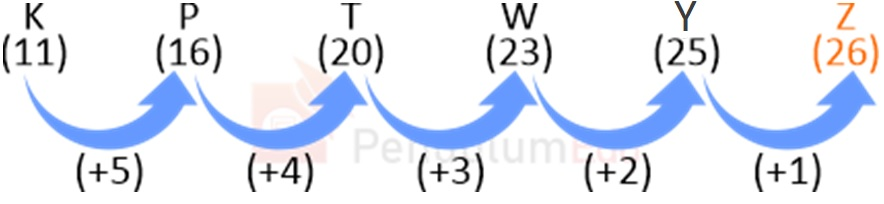Question of The Day26-07-2021

Select the letter that will come next in the following series

K, P, T, W, Y, ?

Correct Answer : d ) Z

Explanation :

According to the question

Let us assume A is numbered as 1, B as 2 and so on till Z being numbered as 26

The common logic applied here isThus, Z is will replace “?”

Hence, (d) is the correct answer.0